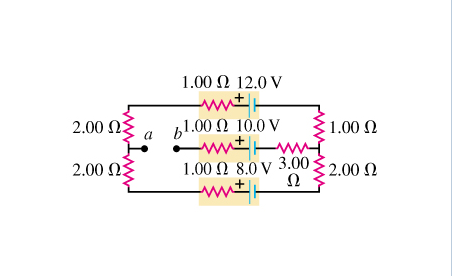# Problem: Part A. Find the potential of point a with respect to point b in the figure. Express your answer using two significant figures.Part B. If points a and b are connected by a wire with negligible resistance, find the magnitude of the current in the 10.0 V battery.  Express your answer using two significant figures. Express your answer using two significant figures.

###### FREE Expert Solution

Kirchhoff's loop rule:

$\overline{){\mathbf{\Sigma }}{\mathbf{V}}{\mathbf{=}}{\mathbf{0}}}$

But:

$\overline{){\mathbf{V}}{\mathbf{=}}{\mathbf{i}}{\mathbf{R}}}$

Part A

No current flows in the middle wire because it is not connected.

The current outside the loop can be taken to be i in a counterclockwise direction.

97% (279 ratings)###### Problem DetailsPart A. Find the potential of point a with respect to point in the figure. Express your answer using two significant figures.

Part B. If points a and b are connected by a wire with negligible resistance, find the magnitude of the current in the 10.0 V battery.  Express your answer using two significant figures. Express your answer using two significant figures.